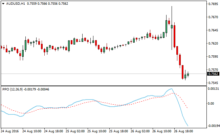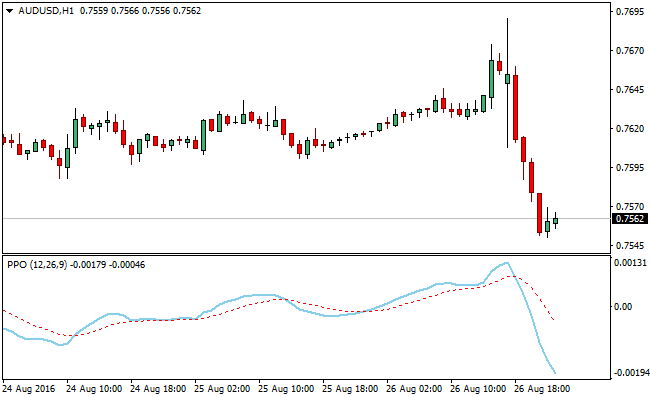# Percentage Price Oscillator (PPO) Momentum Forex IndicatorThe Percentage Price Oscillator (PPO) is a momentum oscillator that gauges the relationship between two moving averages.

To compute the PPO, we subtract the 26-day exponential moving average (EMA) from the 9-day exponential moving average, and the difference is divided by the 26-day exponential moving average.

What we get is a percentage that reveal where the short term average is relative to the longer-term average.

Example ChartThe PPO is able to reveal the convergence and divergence of two moving averages just as seen with the MACD. When the shorter moving average is aligned above the longer moving average the PPO is positive.

Its movement into the positive zone deepens as the gap between shorter moving average and the longer moving average increases. This move is indicative of a strong momentum to the upside.

When the shorter moving average is below the longer moving average the PPO is negative. Its negative reading deepens when the gap between the shorter moving average and the longer moving average increases. This move is indicative of a strong downside momentum.

A high percentage price oscillator (PPO) reading is supposed to encourage traders place buy position(s) when in sync with other signals gotten from other indicators.

In the same vein, a low percentage price oscillator (PPO) reading is meant to drive a bearish sentiment in line with an already exiting sell signal from other source(s).

MT4 Indicator Characteristics

Currency pairs: Any